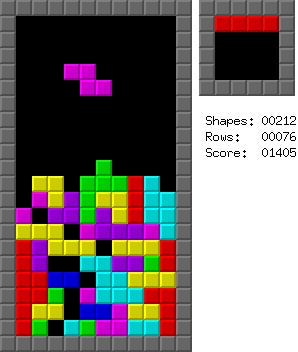# Tetris

A single player game in which the player packs tetrominoes in a rectangular board.## Description

Tetris is an interactive single player game played on a rectangular $n \times m$ board. Initially some of the cells of the board are filled and some are empty. The gameplay is as follows: a tetromino piece is generated at the top of the board and starts falling towards the last row. The player can rotate the piece and slide it horizontally. The fall stops when the piece reaches the last row or lands on an occupied square of the board. The player has a final chance to slide or rotate the piece before it is locked into place and a new tetromino is generated from the top. Whenever a generic $i$-th row of the board is entirely filled by cells of locked pieces, it is cleared. More precisely, each row $j=2, \dots, i$ is replaced by row $j-1$ and row $1$ is replaced by an empty row (rows are indexed from top to bottom, from $1$ to $n$).

The game terminates when a new tetromino can no longer be generated at the top of the board, as at least one of the corresponding cells is already filled.

## Computational complexity

All the following results refer to the version of the game in which only a finite number $p$ of tetrominoes will ever be generated, and the corresponding sequence is known in advance by the player (i.e., it is an input of the problem).

In  it is shown that:

• Maximizing the number of rows cleared is NP-hard is not approximable within a factor $p^{1-\epsilon}$, for any constant $\epsilon > 0$.

• Maximizing the number of tetrominoes placed is not approximable within a factor $p^{1-\epsilon}$, for any constant $\epsilon > 0$.

• Maximizing the number of tetrises, i.e., the simultaneous clearing of four rows is NP-hard.

• Minimizing the height of the highest filled grid cell is not approximable within a factor $2-\epsilon$, for any constant $\epsilon > 0$.

A variant of tetris in which the input pieces can be general $k$-ominoes (i.e., monominoes, dominoes, trominoes, …) has been studied in . The authors consider the problems of deciding whether the player can survive or clear the whole board after all input pieces have been used and show that:

• For $k \ge 4$, both the survival and the clearing problems remain NP-complete (even if the $k$-ominoes cannot be rotated).

• For $k = 3$, the clearing problem is NP-complete (even if the $k$-ominoes cannot be rotated), and the survival problem is NP-complete if rotations are not allowed.

• For $k = 2$, the clearing problem is NP-complete if rotations are not allowed.

• For $k = 1$ rotations are meaningless and both the clearing and the survival problems are in P.

In  it is shown that the clearing problem remains NP-complete even when $m = O(1)$ by a reduction from $3$-partition.

 R. Breukelaar, E. D. Demaine, S. Hohenberger, H. J. Hoogeboom, W. A. Kosters, D. Liben-Nowell, “Tetris is Hard, Even to Approximate”, International Journal of Computational Geometry and Applications, 2004.

 E. D. Demaine, M. L. Demaine, S. Eisenstat, A. Hesterberg, A. Lincoln, J. Lynch, Y. W. Yu, “Total Tetris: Tetris with Monominoes, Dominoes, Trominoes, Pentominoes, …”, Journal of Information Processing, 2017.S. Asif, E. D. Demaine, J. Lynch, M. Singhal, “Tetris is NP-hard even with O(1) columns”, in JCDCGGG 2019.

@misc{cog:tetris,
author = "{CoG contributors}",
title  = "{Tetris --- Complexity of Games}",
year   = "2022",
url    = "https://www.isnphard.com/i/tetris/",
note   = "[Online; accessed 2022-06-02]"
}